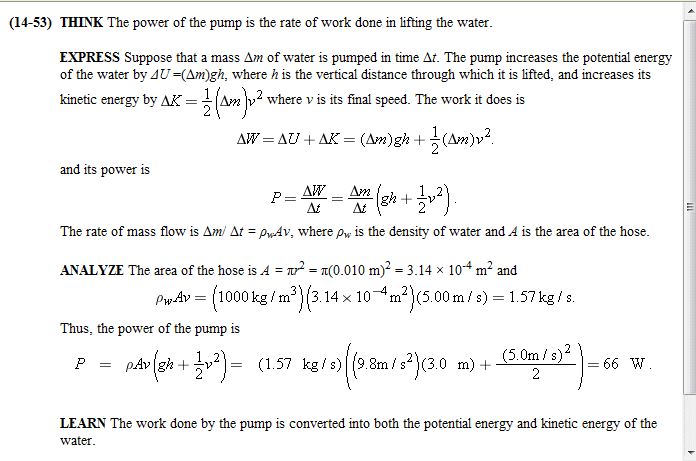# Power of a pump

## Homework Statement

Water is pumped steadily out of a flooded basement at a speed of 5.5 m/s through a uniform hose of radius 1.0 cm. The hose passes out through a window to a street ditch 2.8 m above the waterline. What is the power of the pump?

..

## The Attempt at a Solution

We find the flow which is the area multiplied by the speed. Then we find the power which is the flow x the height (2.8 m) x gravitational acceleration x 1000 (fluid density). At least that's what my friend told me I should do. I had an answer of 47.4129 W but the answer is seemingly wrong. Can someone help me here, please?

I compute 47.4 watts. The problem is not accounting for the length of the hose so there is no hose friction to overcome. I don't see what is wrong with your answer.

Simon Bridge
Homework Helper
There is confusion and uncertainty if you just use equations that you get from someplace without understanding them.

Power is just energy/time

the energy needed to lift a mass m through height h is mgh - like you knew all along.
if it take time T to pump 1kg then the power is P = gh/T
so far so easy?

You are pumping a volume of 550 x pi ccs per second.
1cc of water is 1g, so you are pumping 0.55pi kg of water per second.

this is mass/time ... so we can finesse the previous formula:
P = gh(m/T) = 9.81x2.8x0.55pi = 47.461W

So I agree with LawrenceC - what makes you think this is the wrong answer?

Thank you for the reply and for the explanations.

Well, our instructor posts our homework on wileyplus.com and it gave me an incorrect answer after plugging in the value.

I was checking through the problems on wileyplus and found this method:The values are different, by the way (use the values from the problems statement I posted). I plugged the values and it gave me something around ~55 (or slightly more). The answer was also wrong. I don't think I my calculations are wrong - I triple-checked my computations.

I'm new to fluids as we did not cover it in high school.

Last edited:
Simon Bridge
Homework Helper
Ah - that method also takes account that the pump has to accelerate the water, so gives it some kinetic energy. Your numbers are fine - 55.78J I get.

I think you need to look through your class notes for the definition you are given for the power of a pump. LawrenceC and I (and you, at first) have been working out just the rate of work against gravity alone.

Is there anything left out of the problem you've given to us?
Efficiency of the pump perhaps?

If that is everything then you want to check with other students to see how they did it (did your friend get the right answer?) and, if this is common, confront your instructor: explain how you did it and ask what you missed out.

•Curieuse